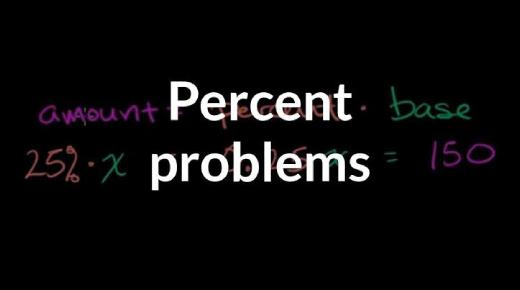# TABE: Math Trivia Quiz On Percent Equation!

12 Questions | Total Attempts: 166SettingsAre you looking for a TABE math trivia quiz on percent equation? If so, you are in luck as the quiz below is perfect for solving any issues you may be having when it comes to math problems that involve percentages. How about you give it a try and get to see how much practice you may need to perfect your skills.

• 1.
150% of 40
• 2.
50% of 27
• 3.
71% of 90
• 4.
10% of 30
• 5.
30% of ____ =18
• 6.
190% of ___ =57
• 7.
30% of ___ =18
• 8.
80% of ___ =64
• 9.
____% of 160=144
• 10.
____% of 150=75
• 11.
___% of 90=63
• 12.
___% of 30=18
Related TopicsBack to top